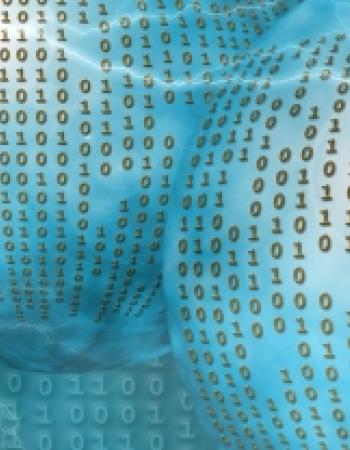# Sumayah Mansour Binhazzaa

Lecturer

Faculty

كلية العلوم
Bulding 5, Third floor, Office 344
sbinhazzaa
course

# 225 Math (Ordinary Differential Equations)

This upper level course provides an introduction to the theory, solution and application of ordinary differential equations. Topics discussed in the course include methods of solving first-order differential equations, higher-order differential equations, modeling with first-order and higher-order differential equations, series solution of linear equations, systems of linear first order differential equations, and numerical solutions of ordinary differential equations. Applications of differential equations in physics, biology, and economics are presented.

The primary audience for this course is students who wish to concentrate in either mathematics or applied mathematics. Students concentrating in a tangentially related field, such as physics, may also be interested in this course.

Prior to enrolling in this course, students should be fluent in differential and integral Calculus as would be acquired in the first two courses of a three or four course sequence of Calculus.

course attachements